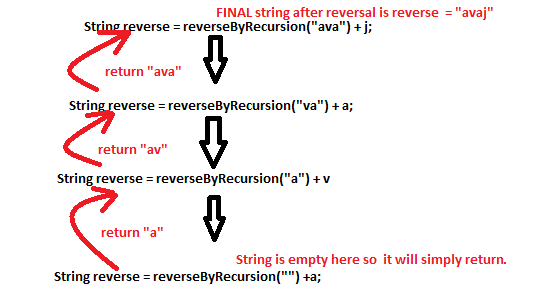## Aug 19, 2013# Reverse a string in java via Iterative and recursive approach.

This is very common interview question asked to warm up the interview session specially recursive approach. This problem can be solved in many ways , we will discuss iterative way first followed by recursive approach.
Iterative approach :
1.  Using normal looping and outcome string holder : It is simplest approach for string reversal .
Algorithm :     Convert Input string into char array
loop char array in opposite direction (from last to first index)
store each character in temporary string holder(outcome string holder.
Sample code and inline comments for above mentioned approach :
``   public StringBuffer reverseString(String str) {       char[] strCharArry = str.toCharArray();  //Convert input string to char array     StringBuffer sbf = new StringBuffer();   // temp string holder to store result     for (int i = strCharArry.length - 1; i >= 0; i--) {         sbf = sbf.append(str.charAt(i));       }       return sbf;     }  ``
we have used StringBuffer(A mutable sister of String) for storing the reversed string.

2. In-place reversal without using extra string holder : In this approach we will stick to normal looping but we will not use any extra string holder. Instead we use some temporary variable to store char and indexes wile iterating.
Algorithm :  Loop input string using two index start and end , until start is less than end
Swap start index char and end index
change the index's position and repeat
Sample code for the above mentioned approach :
``   public String reverseInplace(StringBuffer str) {       char temp;       for (int i = 0, j = str.length() - 1; i < j; i++, j--) {         temp = str.charAt(i);         str.setCharAt(i, str.charAt(j));        str.setCharAt(j, temp);       }       return str.toString();     }  ``
For swapping of char we are using stringBuffer's method to set the char value at specified index.

3. Using XOR operation : It is really tricky one, I admit it. Before going into delve it is worth to  understand the basic concept behind it XOR swapping algorithm.Now using XOR swap algorithm we will solve this problem.
Algorithm :  Loop input string with two index start and end, until start is less than end.
for each char pointing start and end (X = char at start index and Y = char at end index)
do this and swap it
X = X XOR Y
Y = X XOR Y
X = X XOR Y
Now we will write sample code which uses input string as StringBuffer and its method charAt(index) and  in each iteration get char from start and end index and swap it. Sample code is as follows :
``   public StringBuffer reverseByXOROperation(StringBuffer str) {        int inputLenght = str.length();       for (int i = 0, j = inputLenght - 1; i < j; i++, j--) {         str.setCharAt(i, (char)(str.charAt(i) ^ str.charAt(j)));         str.setCharAt(j, (char)(str.charAt(i) ^ str.charAt(j)));         str.setCharAt(i, (char)(str.charAt(i) ^ str.charAt(j)));       }       return str;     }  ``

Recursive approach:
4.Using recursion:  It is most commonly asked approach in an interview.
Algorithm :  Iterate input string fetching one char at a time
repeatedly call recursive method for remaining of the char and append the preprocessed char
Best way to visualize the recursive approach via sample code , so we will see first the sample code :
``   public String reverseByRecursion(String str) {       if (null == str || str.length() == 1) {         return str;       } else {         String reverse = reverseByRecursion(str.substring(1))                        + str.charAt(0);         return reverse;       }     }  ``

How it is working ? : lets take input string as "java"
When first time control comes inside loop , since "java" is not null so if  block is not executed. so it comes in else block, under else  String reverse = reverseByRecursion(str.substring(1)) + str.charAt(0);  changes to String reverse = reverseByRecursion("ava") + j, Diagrammatically have shown below the  recursive call and how it concludes in upward direction.............................................................................................................................................................
Finally reverse is returned to caller of this method as "avaj" and this concludes various string reversal approaches.

Happy learning!!
Nikhil
1.